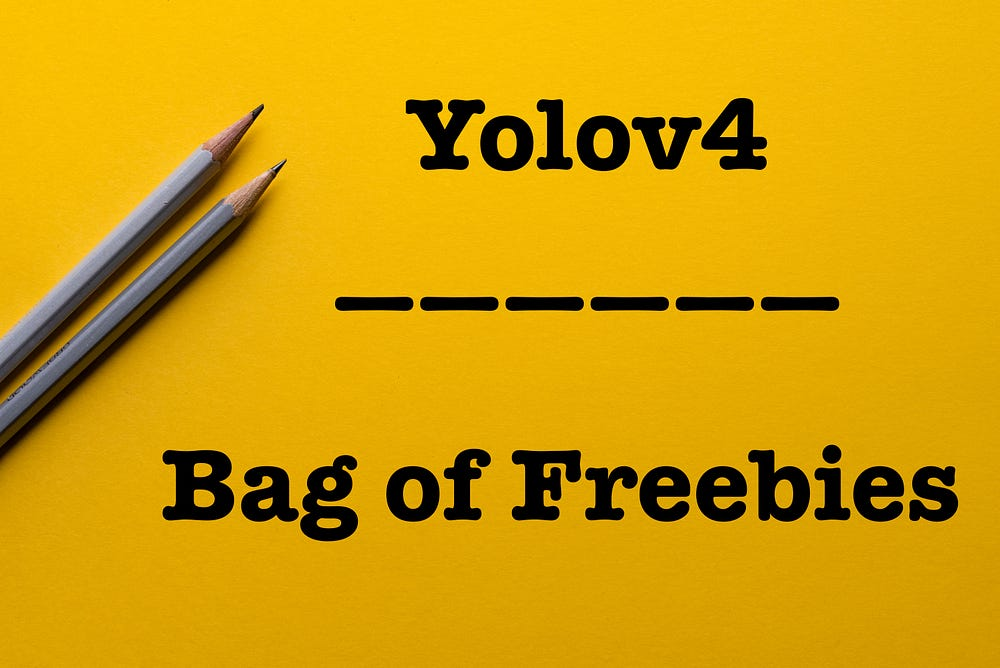# YOLOv4 — Version 1: Bag of Freebies

Original article was published on Deep Learning on Medium# Objective Function of BBox Regression

The last bag of freebies mentioned in the paper is the objective function of Bounding Box (BBox) regression.

What is objective function?

In object detectors, the objective function also called as loss functions are used to penalize and direct the model towards a better convergence at each training step.

Mean Square Error (MSE): The traditional (one should not use) object detector uses MSE to perform regression on center point coordinates and height and width of the BBox i.e. (xc, yc, w, h) or top-left and bottom-right coordinates(tlx, tly, brx, bry).

Anchor based approaches used corresponding offsets to these points.

When we do regression on individual points, it does not take the integrity/coverage area of an object into account.

Furthermore, the loss increases along with scale of the object which is not ideal.

Researchers proposed IoU loss which considers the coverage area of predicted box area and ground truth area.

IOU is scale invariant and thus it does not increase with the scale of box like MSE.

In recent years, there has been improved versions of IOU based loss functions.

## GIoU (Generalized Intersection over Union)

• The original IoU loss only addressed the overlapped bounding boxes and will not provide any learning for the non-overlapping cases. To address this issue, the equation takes into account the non-overlapping cases.
• Where A and B are the prediction and ground truth bounding boxes. C is the smallest convex hull that encloses both A and B.
• The idea of GIoU is to move the predicted box closer to ground truth despite no overlap. In case of simple IoU, it would have simply passed as 0 resulting in no improvement in position of predicted box.
• As the IoU component increases, the value of GIoU converges to IoU.

B : Predicted Box ; Bgt : Ground truth box ; C : Smallest Convex Hull between B and Bgt

## DIoU(Distance-IoU)

• DIoU loss , additionally considers the distance of the center of an object as an extra penalty term.
• The paper introduces an additional penalty term on IoU loss to directly minimize the normalized distance between central points of two bounding boxes, leading to much faster convergence than GIoU loss as shown in fig below.
• The penalty term directly tries to minimize the distance between b which is the center point of the predicted bounding box and bgt which is the center point of the ground truth. They also add denominator c which is the diagonal length of the smallest enclosing box covering the two boxes

## CIoU(Complete IOU)

• CIoU loss , simultaneously considers three metrics: overlapping area, the distance between center points, and the aspect ratio. It is an extension to DIoU loss.

The alpha is a positive trade off parameter where overlaps are given higher priority over non-overlap cases and v gives information about consistency of aspect ratio.

CIoU can achieve better convergence speed and accuracy on the BBox regression problem.

Next Article: YOLOv4 — Version 2: Bag of Specials.